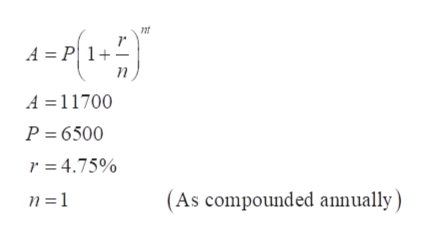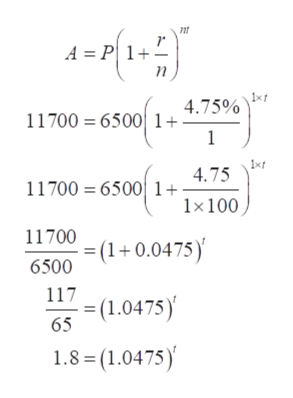# A person invests 6500 dollars in a bank. The bank pays 4.75% interest compounded annually. To the nearest tenth of a year, how long must the person leave the money in the bank until it reaches 11700 dollars?

Question
152 views

A person invests 6500 dollars in a bank. The bank pays 4.75% interest compounded annually. To the nearest tenth of a year, how long must the person leave the money in the bank until it reaches 11700 dollars?

check_circle

Step 1

First of all, consider the formula to calculate compound interest and the terms related to it.

A = the Final Investment Amount in \$

P = the Principal amount of money invested in \$

r = the rate of interest

t = Time in years

n = the number of times interest is compounded per year

As per the given value in the question, get the values of all the terms in the equation.help_outlineImage TranscriptioncloseA = P| 1+ - A =11700 P = 6500 r = 4.75% n =1 (As compounded annually ) fullscreen
Step 2

Now, put the values in the above form...help_outlineImage Transcriptionclosent A = P| 1- 1xt 4.75% 11700 = 6500 1+: 1xf 4.75 11700 = 6500 1+ 1×100 11700 (1+0.0475)' 6500 117 -= (1.0475) 65 1.8 = (1.0475)' fullscreen

### Want to see the full answer?

See Solution

#### Want to see this answer and more?

Solutions are written by subject experts who are available 24/7. Questions are typically answered within 1 hour.*

See Solution
*Response times may vary by subject and question.
Tagged in